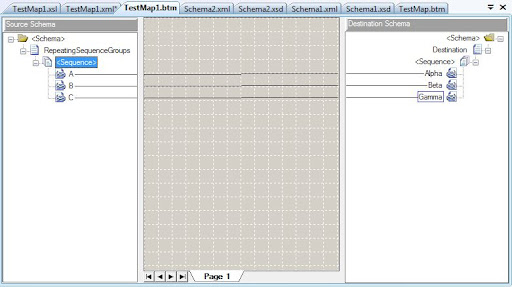Geeks With Blogs
Paul's Petrov Whiteboard [BizTalk, Enterprise Application Integration, Business Process Automation, SOA, .NET]
Repeating sequence groups can often be seen in real life XML documents. It happens when certain sequence of elements repeats in the instance document. Here’s fairly abstract example of schema definition that contains sequence group:
<xs:schemaxmlns:b="http://schemas.microsoft.com/BizTalk/2003"
xmlns:xs="http://www.w3.org/2001/XMLSchema"
xmlns="NS-Schema1"
targetNamespace="NS-Schema1" >
<xs:elementname="RepeatingSequenceGroups">
<xs:complexType>
<xs:sequencemaxOccurs="1"minOccurs="0">
<xs:sequencemaxOccurs="unbounded">
<xs:elementname="A"type="xs:string" />
<xs:elementname="B"type="xs:string" />
<xs:elementname="C"type="xs:string"minOccurs="0" />
</xs:sequence>
</xs:sequence>
</xs:complexType>
</xs:element>
</xs:schema>
And here’s corresponding XML instance document:
<ns0:RepeatingSequenceGroupsxmlns:ns0="NS-Schema1">
<A>A1</A>
<B>B1</B>
<C>C1</C>
<A>A2</A>
<B>B2</B>
<A>A3</A>
<B>B3</B>
<C>C3</C>
</ns0:RepeatingSequenceGroups>
As you can see elements A, B, and C are children of anonymous xs:sequence element which in turn can be repeated N times. Let’s say we need do simple mapping to the schema with similar structure but with different element names:
<ns0:Destinationxmlns:ns0="NS-Schema2">
<Alpha>A1</Alpha>
<Beta>B1</Beta>
<Gamma>C1</Gamma>
<Alpha>A2</Alpha>
<Beta>B2</Beta>
<Gamma>C2</Gamma>
</ns0:Destination>
The basic map for such typical task would look pretty straightforward:If we test this map without any modification it will produce following result:
<ns0:Destinationxmlns:ns0="NS-Schema2">
<Alpha>A1</Alpha>
<Alpha>A2</Alpha>
<Alpha>A3</Alpha>
<Beta>B1</Beta>
<Beta>B2</Beta>
<Beta>B3</Beta>
<Gamma>C1</Gamma>
<Gamma>C3</Gamma>
</ns0:Destination>
The original order of the elements inside sequence is lost and that’s not what we want. Default behavior of the BizTalk 2009 and 2010 Map Editor is to generate compatible map with older versions that did not have ability to preserve sequence order. To enable this feature simply open map file (*.btm) in text/xml editor and find attribute PreserveSequenceOrder of the root <mapsource> element. Set its value to Yes and re-test the map:
<ns0:Destinationxmlns:ns0="NS-Schema2">
<Alpha>A1</Alpha>
<Beta>B1</Beta>
<Gamma>C1</Gamma>
<Alpha>A2</Alpha>
<Beta>B2</Beta>
<Alpha>A3</Alpha>
<Beta>B3</Beta>
<Gamma>C3</Gamma>
</ns0:Destination>
The result is as expected – all corresponding elements are in the same order as in the source document. Under the hood it is achieved by using one common xsl:for-each statement that pulls all elements in original order (rather than using individual for-each statement per element name in default mode) and xsl:if statements to test current element in the loop:
<xsl:templatematch="/s0:RepeatingSequenceGroups">
<ns0:Destination>
<xsl:for-eachselect="A|B|C">
<xsl:iftest="local-name()='A'">
<Alpha>
<xsl:value-ofselect="./text()" />
</Alpha>
</xsl:if>
<xsl:iftest="local-name()='B'">
<Beta>
<xsl:value-ofselect="./text()" />
</Beta>
</xsl:if>
<xsl:iftest="local-name()='C'">
<Gamma>
<xsl:value-ofselect="./text()" />
</Gamma>
</xsl:if>
</xsl:for-each>
</ns0:Destination>
</xsl:template>
BizTalk Map editor became smarter so learn and use this lesser known feature of XSLT 2.0 in your maps and XSL stylesheets.